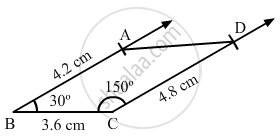Share

# Construct a Quadrilateral Abcd, Where Ab = 4.2 Cm, Bc = 3.6 Cm, Cd = 4.8 Cm, ∠B = 30° and ∠C = 150°. - Mathematics

Course
ConceptConstructing a Quadrilateral When Two Adjacent Sides and Three Angles Are Known

#### Question

Construct a quadrilateral ABCD, where AB = 4.2 cm, BC = 3.6 cm, CD = 4.8 cm, ∠B = 30° and ∠C = 150°.

#### Solution

Steps of construction:

Step I: Draw BC = 3 . 6 cm .

Step II: Construct ∠ABC = 30° at B .

Step III : With B as the centre and radius 4 . 2 cm, cut off BA = 4 . 2 cm .

Step IV: Construct ∠BCD = 150° at C .

Step V : With C as the centre and radius 4 . 8 cm, cut off CD = 4 . 8 cm .